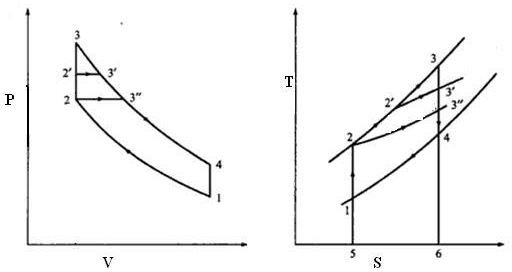# Marine Diesel engine cycles compared – Otto cycle and other cycles

## Introduction

In the last three articles we studied about Otto cycle, diesel cycle and dual cycle and looked at their thermal efficiency. In this article we will take a collective look at these three cycles in order to compare and contrast them, so that we can come to know the relative advantages and disadvantages of these cycles.

## The 3 Cycles Compared

In the last article we compared the three cycles their compression ratio and heat addition were kept constant for all cycles. In this article we will focus on constant compression ratio but which is same as last comparison, but the difference would be that the constant heat rejection would be calculated instead of constant head addition. The P-V and T-S diagrams of these three cycles for such a situation are drawn simultaneously as described below.In the above diagrams the following are the cycles

• Otto cycle: 1 – 2 – 3 – 4 – 1
• Dual cycle: 1 – 2 – 2’ – 3’ – 4 – 1
• Diesel cycle: 1 – 2 – 3” – 4 – 1

Remember that we are assuming constant compression ratio for all three cycles which is given by V1/V2

The other parameter which is constant is the heat rejected from the cycle which is given by the following in each case as per the T-S diagram

All cycles: Area under 4 – 1 – 5 – 6 in the T-S diagram

The heat supplied is different in each case and can be established from the T-S diagram as follows

• Otto cycle: Area under 2 – 3 – 6 – 5 say q1
• Dual cycle: Area under 2 – 2’ – 3’ – 6 – 5 say q2
• Diesel cycle: Area under 2 – 3” – 6 – 5 say q3

It can also be seen from the same diagram that q3<q2<q1

We know that thermal efficiency is given by 1 – heat rejected/heat supplied

Since heat rejected is same and we know the order of magnitude of heat supplied, we can combine this information to conclude that

Thermal efficiency of these engines under given circumstances is of the following order

Otto>Dual>Diesel

Hence we see that in this case as well the Otto cycle shows higher thermal efficiency than a dual cycle and even better than the diesel cycle. We will study another combination in the next article.